# Principal and interest

Peter put € 270 principal into bank and at the end of the year on account was € 282. To what annual interest rate that Peter had deposited money in the bank?

p =  4.44 %

### Step-by-step explanation:Did you find an error or inaccuracy? Feel free to write us. Thank you!Tips to related online calculators
Do you want to convert time units like minutes to seconds?

## Related math problems and questions:

• Simple interest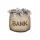Peter put into bank 853 euros deposit. After 7 years on account overall was 984 euro. What was the interest rate if bank add simple interest?
• Combined interest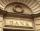Combined interest: Carol has deposited CZK 100,000 in the bank with an annual interest rate of 1.5%. The money was put into the account 5.5.2016 and withdraw them all before Christmas 20.12.2016. How much money did she withdraw?
• Compound interestCompound interest: Clara deposited CZK 100,000 in the bank with an annual interest rate of 1.5%. Both money and interest remain deposited in the bank. How many CZK will be in the bank after 3 years?
• Deposit 3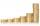After a year, Martin had on bank account € 2028. What was the interest rate when the initial deposit was € 1879?
• Mr. Brown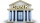Mr. Brown invested 15,000 euros in the bank for an annual term deposit. After the first year, he gained 600 euros. What was the annual interest rate of his deposit?
• Annual pensionCalculate the amount of money generating an annual pension of EUR 1000, payable at the end of the year and for a period of 10 years, shall be inserted into the bank to account with an annual interest rate of 2%
• DepositOh I total of \$15,000 deposited into simple interest accounts the annual simple interest rate on one account at 6% the annual simple interest rate on the second account at 7% how much should be invested in each account so that the total interest earned is
• 3 years savingsSimple interest problem. Tereza has deposited CZK 100,000 in the bank with an annual interest rate of 1.5%. Interest is added each year and left aside. How many CZK (crowns) will have total after 3 years?
• Deposit is pesosSally deposits Php.22,000 in her savings account. If the bank pays 1.5% interest per year, how much will she receive at the end of the year?
• Bank account CZWe will deposit CZK 6,600 into the bank for 1 year with an interest rate of 2.5%. The interest tax is 15%. How many crowns will we receive after one year?
• Mr. Vojta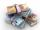Mr. Vojta put in the bank 35000 Kč. After a year bank, he credited with an interest rate of 2% of the deposit amount. How then will Mr. Vojta in the bank?
• Millionaire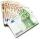Mr. Smith have gone withdraw from bank saving interest € 1,500. How big was his initial deposit if the annual interest rate is 1.5%?
• Two accounts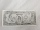Two accounts in the bank, one per year interest 2%, the second 3%. Total interest income 1900 USD. If interest rates were reversed, the yield would be USD 200 higher. What are the amounts on each account?
• SavingsThe depositor regularly wants to invest the same amount of money in the financial institution at the beginning of the year and wants to save 10,000 euros at the end of the tenth year. What amount should he deposit if the annual interest rate for the annua
• Bank deposit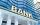Ms Jana after the withdraw amount of 2,500 euros after year saving in bank. What was her deposit if the interest rate was 2.5% p.a..
• BankPaul put 10000 in the bank for 6 years. Calculate how much you will have in the bank if he not pick earned interest or change deposit conditions. The annual interest rate is 3.5%, and the tax on interest is 10%.
• When will I be a millionaire?Barry monthly send 280 euros to the bank, which he deposits bear interest of 2.1% p. A. Calculate how many months must Barry save to save 1000000 euros? Inflation, interest rate changes, or bank failures are ignored.